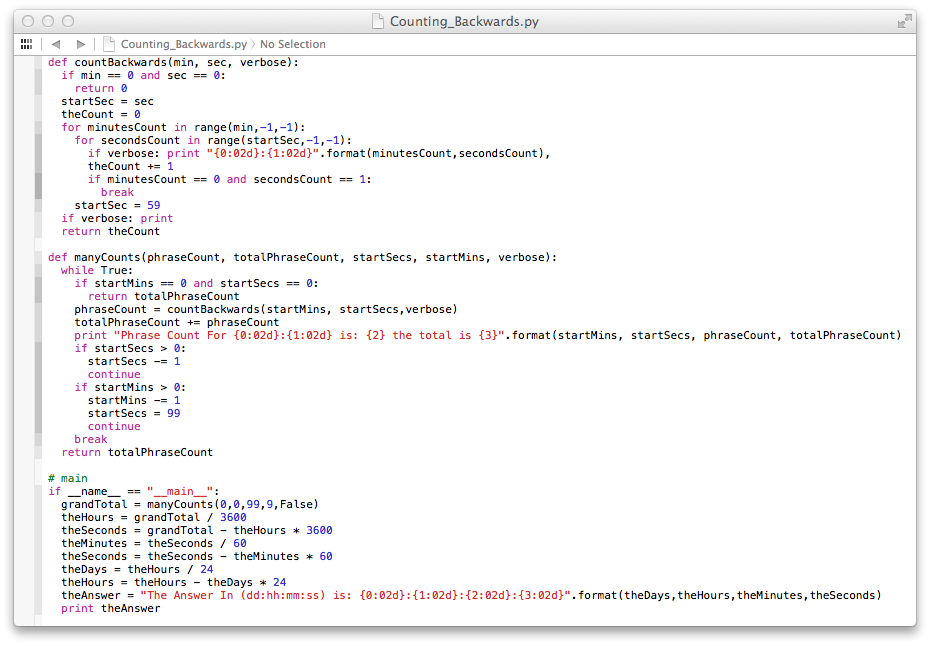## Wednesday, March 12, 2014

### How Many Numbers Do You Speak

How Many Numbers Do You Speak When Counting Backwards?
or
Can Someone Speak For
(dd:hh:mm:ss) is: 03:16:45:00 (correction: see below)

Note:  The motivation for this post can be found at the following link: "http://www.youtube.com/user/vihartvihart".  Of course I do not know how many videos will be eventually posted but I can imagine that there will be 999 videos with this same topic. This post was written the same day 9:91 was posted.

Suppose you were to speak the numbers backwards from 99 down to 1, how many numbers would you speak?  The answer seems pretty easy: The following set when spoken aloud yields ninety-nine numbers: {99, 98, 97, ..., 10 , 9, 8, 7, 6, 5, 4, 3, 2, 1}.

Now suppose you are looking at a microwave oven timer and you enter 1:99.  This is taken by the microwave software as the input one minute and ninety-nine seconds.  This gives the following set of numbers: {199, 198, 197, ..., 110, 109, 108, 107, 106, 105, 104, 013, 102, 101, 100, 59, 58, 57, 56, ..., 10, 9, 8, 7, 6, 5, 4, 3, 2, 1}.

Notice when the count reaches 100 it then continues with 59, 58, 57, down to 1. so entering 199 in a microwave only yields 99+60 or 159 numbers. In a similar manner entering 299 yields 99+60+60 or 219 numbers.

Now suppose you enter 9:99 how many numbers do you get?

And finally suppose you enter 9:99 and count backwards. Next, at the end you enter 9:98 and count backward, then at the end you enter 9:97 and so forth all the way to 0:99, 0:98, 0:97, ...,  0:05, 0:04, 0:03, 0:02, 0:01.

What is the total?

Below is a python app that, I think, computes the number of numbers.  The grand total is very large.The Answer
A much shorter way to say all the numbers is to understand that once you have spoken a number you no longer need to speak it again you can just refer to the one already spoken.  This is in the best tradition of Mathematics.  Once something is done then it stays done!

The total number of spoken numbers remains the same but we can organize them for reuse.

For example:  the set {0:99, 0:98, 0:97, ..., 0:03, 0:02, 0:01 } contains the set {0:98, 0:97, ..., 0:03, 0:02, 0:01} and so forth all the way down to the set {0:03, 0:02, 0:01) and finally the set {0:01}.

When we apply this to speaking the numbers displayed on the microwave we get the following kinds of sets.

The 39 Second Sets:  These are the numbers from, for example:

9:99 down to 9:60, or
8:99 down to 8:60, and
so on down to
0:99 down to 0:60.

There are 10 of these sets. And we see they take up 390 seconds total.

The 60 Second Sets:  These are the numbers from, for example:
9:59 down to 9:00, or
8:59 down to 8:00, and
so on down to
1:59 down to 1:00 and finally
0:59 down to 0:01.

There are 10 of these sets and they take up 590 seconds total.

Adding these numbers together we get 989 seconds or 16 minutes and 29 seconds.